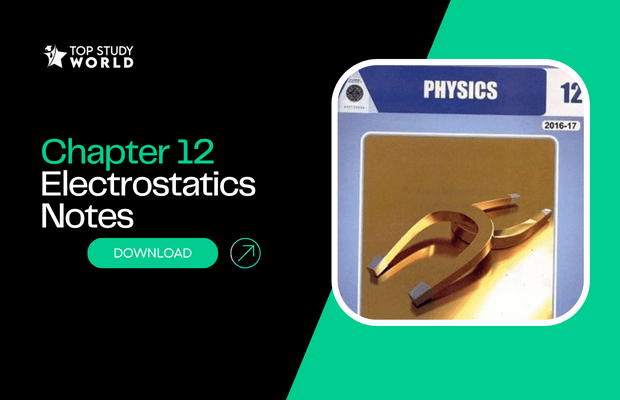Are you finding the F.Sc Part 2 Physics notes for the chapter 12 having the solution to exercise short questions, numerical problems and theory to the point? You are in the right place. The best part! You will get the download link to the PDF at no cost. You can also print the PDF if you want. Do you know?

These notes have the solution of numerical problems, solved exercise short questions and easy wording theory all in one PDF.

These easy notes cover the following topics:

• Introduction
• Kinds of charges
• Electric Force
• Quantitative nature of Electric force
• Coulomb`s Law
• Statement
• Mathematical form
• Vector form
• Effect of medium
• Fields of force
• Electric field lines
• Direction of electric field
• Strength of electric field
• Uniform electric field
• Properties of electric field lines
• Application of electrostatics
• Ink jet Printers
• Electric Flux
• Electric Flux through a surface enclosing a charge
• Gauss`s Law
• Applications of Gauss`s Law
• Electric Potential
• Absolute Electric potential
• Electron volt
• Comparison
• Capacitor
• Capacitance
• Electric Polarization
• Charging and discharging of capacitor

## Chapter 12: Electrostatic Notes PDF

• PDF Details
• Size: 3.11 MB | Pages: 61 |
• Content: Short Questions, Numerical Problems, Theory

## Need Notes for All the Chapters of 2nd Year Physics In One Place?

No matters what board you belong to, notes are beneficial for:

• DG Khan Board